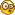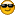I suspected with a size of Spherical Area

• 3 Replies
• 342 ViewsDanang

• 2766
• Phew FE Map is under construction. #DisclaimerI suspected with a size of Spherical Area
« on: January 06, 2019, 09:05:57 PM »
In fact the "6" was shortened by 0.79289 to give the same 11.9633. Let's see
TRY: Phew = 3.17157 and (Curved Grided) South Pole Centered FE AKA Phew FE ~Danang

• 2766
• Phew FE Map is under construction. #DisclaimerRe: I suspected with a size of Spherical Area
« Reply #1 on: January 06, 2019, 09:41:40 PM »
IF the cylinder's height and radius both are substracted with phew ratio of 0.79289, and then this new sized cylinder's two base areas + cylinder's surface area equals a sphere's AREA with radius unchanged, i.e. 1.

Half Sphere Area (for r=1) = Cylinder's Base Area (for r=0.79289 and h=0.79289) + Cylinder's Surface Area (for r=0.79289 and h=0.79289) = r^2 × Phew + (r × 2 Phew) × h =  0.79289^2 × 3.17157 + (0.79289 × 2 × 3.17157) × 0.79289 = 5.9816
Full sphere = 2 × 5.9816 = 11.9633« Last Edit: January 06, 2019, 09:46:26 PM by Danang »
TRY: Phew = 3.17157 and (Curved Grided) South Pole Centered FE AKA Phew FE ~Danang

• 2766
• Phew FE Map is under construction. #DisclaimerRe: I suspected with a size of Spherical Area
« Reply #2 on: January 06, 2019, 09:53:00 PM »
The ratio of Spherical Area : its Frame = 11.9633 : 24 = 0.49847.

It's similar to the ratio of a Spherical Volume : its Frame = 3.9878 : 8 = 0.49847TRY: Phew = 3.17157 and (Curved Grided) South Pole Centered FE AKA Phew FE ~Danang

• 2766
• Phew FE Map is under construction. #DisclaimerRe: I suspected with a size of Spherical Area
« Reply #3 on: January 14, 2019, 06:17:49 AM »
Too much Youtube I guess.NOWAY..  The calculation will get back to old concept:

The material for spherical area is a cylinder with l = C = 6.34314 and h or w = 1/2C = 3.17157.
And then the height and length gets curved and lost 0.2071 part each.

sA = C × 1/2 C × 0.79289 × r² = 6.34314 × 3.17157 × 0.79289² = 12.6475 r².

That's it.
« Last Edit: January 14, 2019, 11:32:20 PM by Danang »
TRY: Phew = 3.17157 and (Curved Grided) South Pole Centered FE AKA Phew FE ~